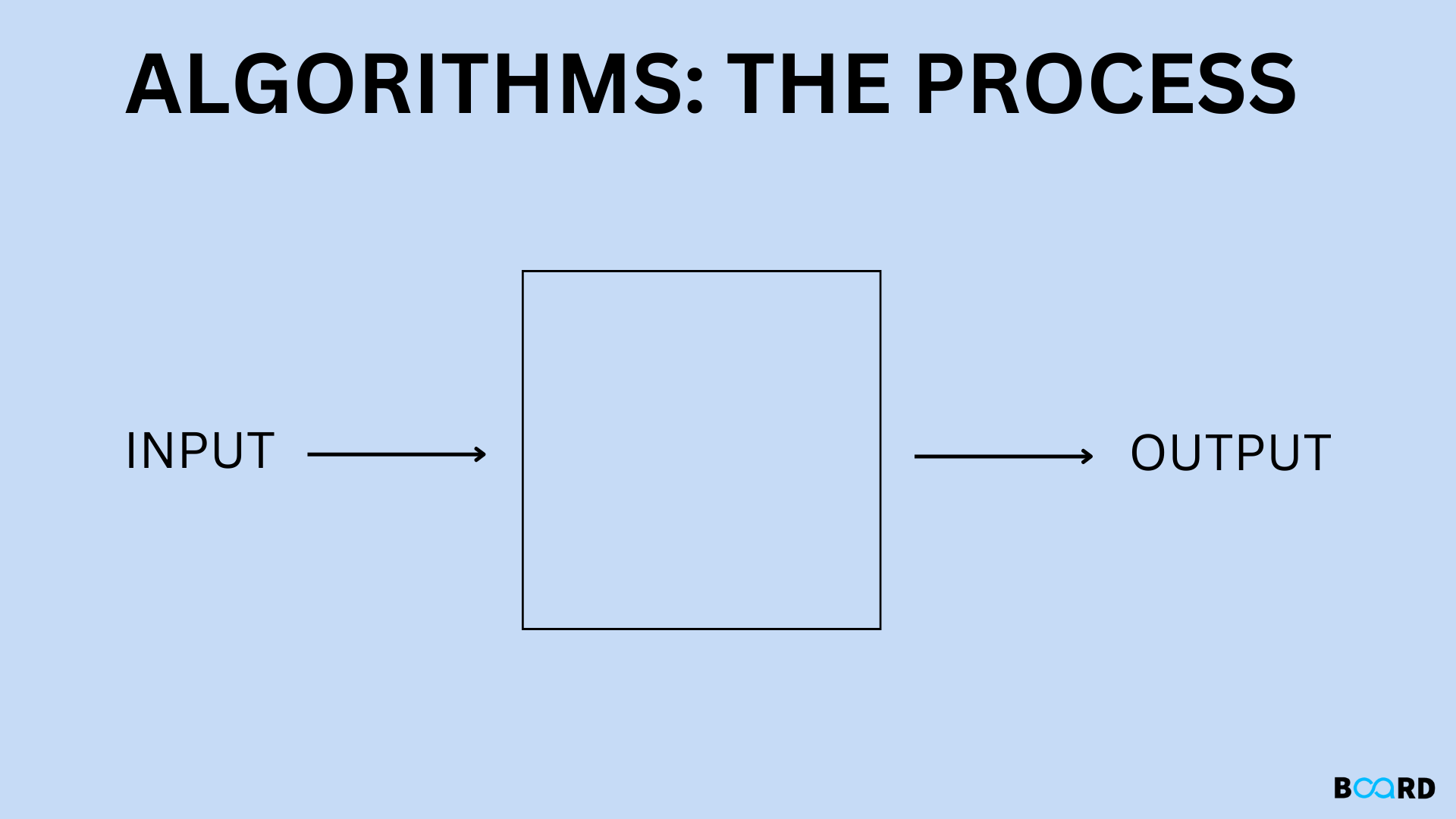# What Is An Algorithm (Understanding The Ingredients)### Introduction

An algorithm is just a step-by-step process that specifies a series of directives that must be followed in a particular sequence in order to yield the desired outcome. An algorithm may be implemented in more than one programming language because algorithms are typically developed independently of the underlying languages. A few characteristics of an algorithm include clarity, excellence, effectiveness, and language independence. An algorithm's performance and scalability are what really determine how important it is.

### Elements of Algorithm

A set of instructions called an algorithm is what a computer must follow in order to perform computation or other problem-solving tasks.

• A formal definition of an algorithm states that it is a bounded set of instructions that are carried out in a particular order to complete a specific task.
• Simple logic to a problem is introduced as an impromptu characterization in the form of a flowchart or pseudocode; it is not the entire programme or code.
• Input: A formula needs some input variables. A value other than 0 may be provided as input to an algorithm.
• Output: An algorithm will produce one or more results after it runs.
• Unambiguity: Unambiguous is the definition of a perfect algorithm, so its directives must be unambiguous and simple.
• Finiteness:A finite algorithm is required. In this context, "finiteness" refers to the requirement that an algorithm have a finite number of instructions, or instructions that can be counted.
• Effectiveness: An algorithm's instructions should be sufficient because they have an impact on the entire process.
• Language independence: A language-independent algorithm is one whose instructions can be incorporated in any language and yield the same outcomes.

### Algorithm Types

• Brute Force Algorithm

This algorithm was created using the general logic structure. It is also known as an exhaustive search algorithm because it explores every avenue before delivering the needed response.

• Dynamic Programming

By storing interim results, it increases the algorithm's effectiveness. To find the ideal response to the issue, five steps are taken:

1. To find the optimal way, it breaks the problem down into smaller problems. It divides the issue into smaller issues and then chooses the best answer from those smaller issues.
2. The act of memoizing involves keeping track of the solutions to smaller problems. Reuse the outcome to avoid having to compute it again for the identical subproblems.
3. It then calculates the output of the sophisticated programme.
• Divide and Conquer

The implementation of this algorithm is simple. It makes it possible for you to build algorithms piecemeal. It disassembles the algorithm in order to find various solutions to the issue. It enables you to break the issue up into different approaches, producing legitimate output from legitimate input. This precise output is passed on to another process.

• Backtracking

If the solution does not adhere to the problem's constraints, the algorithmic process recursively discards it. You will now examine algorithm analysis after having a solid understanding of what an algorithm is and its methods.

• Branch and Bound Algorithm

The bound and branch algorithm can only be used to resolve integer programming issues. All sets of viable solutions are split up into smaller subsets using this technique. The best answer is then determined by further evaluating these subsets.

• Randomized Algorithm

You have input and output that are predetermined, just like in a typical algorithm. Deterministic algorithms follow predefined steps and have a defined set of inputs and outputs. Compared to non-deterministic algorithms, they are more effective.

• Greedy Algorithm

This algorithm paradigm aims to select the best solution by making the best decision at each iteration. It takes less time to execute and is easy to set up. However, it is only the best option in a very small number of circumstances.

### Algorithm Complexity

The algorithm's performance can be measured in two ways:

Time Complexity

Time complexity is the length of time needed to complete an algorithm's execution. The time complexity of an algorithm is expressed using the big O notation. In this instance, big O notation is the asymptotic notation used to describe time complexity. The steps needed to complete the execution are counted in order to determine the time complexity. Let's examine a time complexity illustration.

Space Complexity

The space complexity of an algorithm refers to how much space is needed for it to solve a problem and generate an output. Big O notation is used to express space complexity as well as time complexity. For the following reasons, the algorithm needs the space to store:

1. Instructions for the program.
2. Constant values.
3. Variable values.
4. Function calls, jumping statements, and so on.

Space Complexity = Auxiliary Space + Input Size

### Algorithm 1: Find Sum of Two Numbers

Step 1: Start

Step 2: Declare sum, num1, num2 variables.

Step 3: Read values num1 and num2.

Step 4: Add num1 and num2 and assign the result to sum.

sum←num1+num2

Step 5: Display sum

Step 6: Stop

### Algorithm 2: Prime Number Verification

Step 1: Start

Step 2: Declare variables n, i, flag.

Step 3: Initialize variables

flag ← 1

i ← 2

Step 4: Read n from the user.

Step 5: Repeat the steps until i=(n/2)

5.1 If remainder of n÷i equals 0

flag ← 0

Go to step 6

5.2 i ← i+1

Step 6: If flag = 0

Display n is not prime

else

Display n is prime

Step 7: Stop